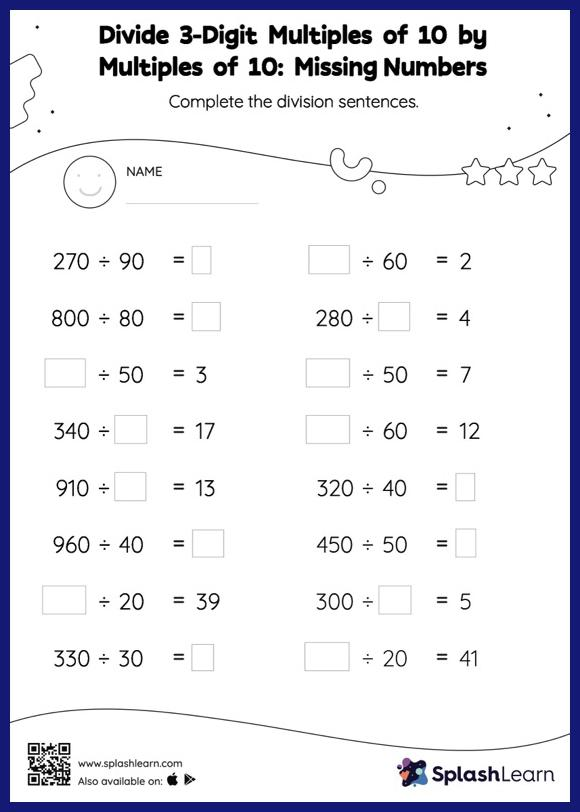# Divide 3-Digit Multiples of 10 by Multiples of 10: Missing Numbers Worksheet

Home > Divide 3-Digit Multiples of 10 by Multiples of 10: Missing NumbersThis divide 3-digit multiples of 10 by multiples of 10 worksheet consists of tasks to help your young mathematician develop fluency with division. When dividing by 10 or its multiples, students look at the number of zeros at the end of the dividend or divisor to determine the quotient. They use the same understanding to find the missing number in divide 3-digit multiples of 10 by multiples of 10 worksheet.